# NCERT Solutions for Class 6 Maths Chapter 8 Decimals Ex 8.1

NCERT Solutions for Class 6 Maths Chapter 8 Decimals Ex 8.1 are part of NCERT Solutions for Class 6 Maths. Here we have given NCERT Solutions for Class 6 Maths Chapter 8 Decimals Ex 8.1.

 Board CBSE Textbook NCERT Class Class 6 Subject Maths Chapter Chapter 8 Chapter Name Decimals Exercise Ex 8.1 Number of Questions Solved 10 Category NCERT Solutions

## NCERT Solutions for Class 6 Maths Chapter 8 Decimals Ex 8.1

Question 1.
Write the following as numbers in the given table:
(a)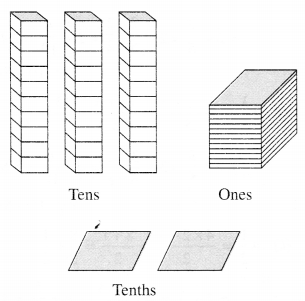(b)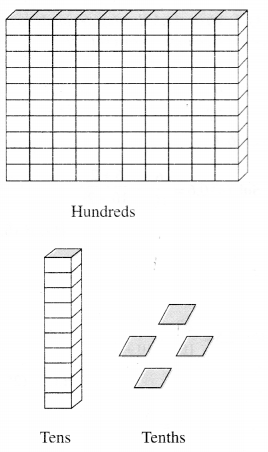Hundreds (100) Tens (10) Ones (1) Tenths ($$\frac { 1 }{ 10 }$$)

Solution.

 Hundreds (100) Tens (10) Ones (1) Tenths ($$\frac { 1 }{ 10 }$$) (a) 0 3 1 2 (b) 1 1 0 4

Question 2.
Write the following decimals in the place value table :
(a)
19.4
(b)
0.3
(c)
10.6
(d)
205.9
Solution.

 Hundreds Tens Ones Tenths (a) 0 1 9 4 (b) 0 0 0 3 (c) 0 1 0 6 (d) 2 0 5 9

Question 3.
Write each of the following as decimals:
(a)
Seven-tenths
(b) Two tens and nine-tenths
(c) Fourteen point six
(d) One hundred and two ones
(e) Six hundred point eight
Solution.
(a) 7 tenths : $$\frac { 7 }{ 10 }$$ =0.7.
(b) Two tens and nine-tenths = 20 + $$\frac { 9 }{ 10 }$$  =20.9
(c) Fourteen point six = 14.6
(d) One hundred and two-ones =100 + 2=102
(e) Six hundred point eight = 600.8

Question 4.
Write each of the following as decimals:
(a)
$$\frac { 5 }{ 10 }$$
(b) 3 +$$\frac { 7 }{ 10 }$$
(c) 200+60+5+$$\frac { 1 }{ 10 }$$
(d) 70+$$\frac { 8 }{ 10 }$$
(e) $$\frac { 2 }{ 10 }$$
(g) $$\frac { 3 }{ 2 }$$
(h) $$\frac { 2 }{ 5 }$$
(i) $$\frac { 12 }{ 5 }$$
(j) $$\frac { 3 }{ 5 }$$
(k) 4$$\frac { 1 }{ 2 }$$
Solution.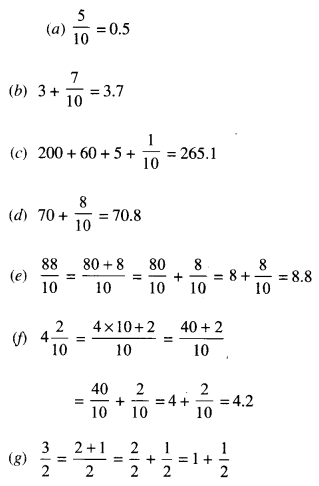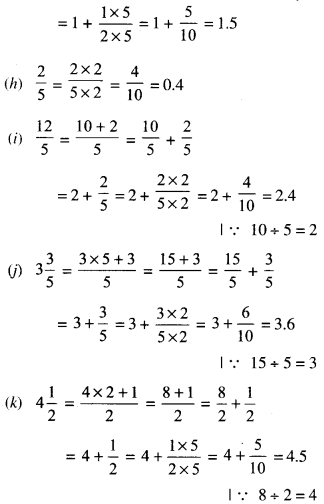Question 5.
Write the following decimals as fractions. Reduce the fractions to lowest form :
(a) 0.6
(b) 205
(c) 1.0
(d) 3.8
(e) 13.7
(f) 21.2
(g) 6.4
Solution.
(a)
$$0.6=\frac { 6 }{ 10 } =\frac { 6\div 2 }{ 10\div 5 }$$
∵  H.C.F.(6,10) = 2
= $$\frac { 3 }{ 5 }$$

(b)
$$2.5=\frac { 25 }{ 10 } =\frac { 25\div 5 }{ 10\div 5 }$$
∵  H.C.F.(25,10) = 5
= $$\frac { 5 }{ 2 }$$

(c)
$$1.5=\frac { 10 }{ 10 } =\frac { 10\div 10 }{ 10\div 10 }$$
∵  H.C.F.(10,10) = 10
= $$\frac { 1 }{ 1 }$$ =1

(d)
$$3.8 =\frac { 38 }{ 10 } =\frac { 38\div 2 }{ 10\div 2 }$$
∵  H.C.F.(38,10) = 2
= $$\frac { 19 }{ 5 }$$

(e)
$$13.7=\frac { 137 }{ 10 }$$

(f)
$$21.2=\frac { 212 }{ 10 } =\frac { 212\div 2 }{ 10\div 5 }$$
∵  H.C.F.(212,10) = 5
= $$\frac { 106 }{ 5 }$$

(g)
$$6.4=\frac { 64 }{ 10 } =\frac { 64\div 2 }{ 10\div 5 }$$
∵  H.C.F.(64,10) = 2
= $$\frac { 32 }{ 5 }$$

Question 6.
Express the following as cm using decimals:
(a)
2 mm
(b)
30 mm
(c)
116 mm
(d)
4cm 2mm
(e)
11cm 52mm
(f)
83 mm
Solution.
(a)
$$2mm =\frac { 2 }{ 10 }$$
∵  $$1mm =\frac { 1 }{ 10 }$$
= 0.2cm

(b)
$$30mm =\frac { 30 }{ 10 }$$
∵  $$1mm =\frac { 1 }{ 10 }$$
= 3cm = 3.0cm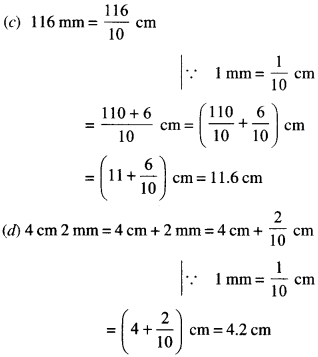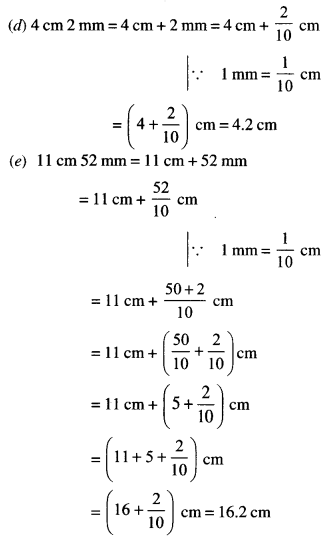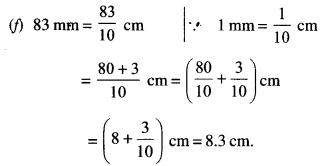Question 7.
Between which two whole numbers on the number line are the given numbers lie? Which of these whole numbers is nearer the number?
(a) 0.8
(b) 5.1
(c) 2.6
(d) 6.4
(e) 9.0
(f) 4.9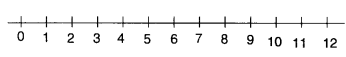Solution.
(a) 0.8. The number 0.8 is between the two whole numbers 0 and 1. The whole number 1 is nearer the number 0.8.

(b) 5.1. The number 5.1 is between the two whole numbers 5 and 6. The whole number 5 is nearer the number 5.1.

(c) 2.6. The number 2,6 is between the two whole numbers 2 and 3. The whole number 3 is nearer the number 2.6.

(d) 6.4 The number 6.4 is between the two whole numbers 6 and 7. The whole number 6 is nearer the number 6.4.

(e) 0. 9.0 itself is a whole number.

(f) 4.9. The number 4.9 is between the two whole numbers 4 and 5. The whole number 5 is nearer the number 4.9.

Question 8.
Show the following numbers on the number line:
(a)
2
(b) 1.9
(c) 1
(d) 2.5.
Solution.
(a) 0.2. We know that 0.2 is more than zero but less than one. There are 2-tenths in it. Divide the unit length between 0 and 1 into 10 equal parts and take 2 parts as shown below: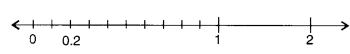(b) 1.9. We know that 1.9 is more than one but less than two. There are 9-tenths in’it. Divide the unit length between 1 and 2 into 10 equal parts and take 9 parts as shown below: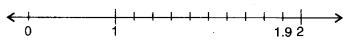(c) 1.1. We know that 1.1 is more than one but less than two. There is one-tenth in it. Divide the unit length between 1 and 2 into 10 equal parts and take 1 part as shown below: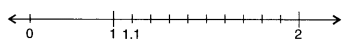(d) 2.5. We know that 2.5 is more than two but less than three. There are 5-tenths in it. Divide the unit length between 2 and 3 into 10 equal parts and take 5 parts as shown below :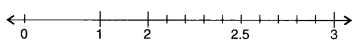Question 9.
Write the decimal number represented by the points A, B, C, D on the given number line :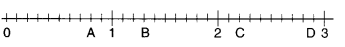Solution.
(i) A. The decimal number represented by the point A is 0.8 as the unit length between 0 and 1 has been divided into 10 equal parts and 8 parts have been taken.

(ii) B. The decimal number represented by the point B is 1.3 as the unit length between 1 and 2 has been divided into 10 equal parts and 3 parts have been taken.

(iii) C. The decimal number represented by the point C is 2.2 as the unit length between 2 and 3 has been divided into 10 equal parts and 2 parts have been taken.

(iv) D. The decimal number represented by the point D is 2.9 as the unit length between 2 and 3 has been divided into 10 equal parts and 9 parts have been taken.

Question 10.
(a) The length of Ramesh’s notebook is 9 cm 5 mm. What will be its length in cm?
(b) The length of a young gram plant is 65 mm. Express its length in cm.
Solution.
(a) Length of Ramesh’s notebook = 9 cm 5mm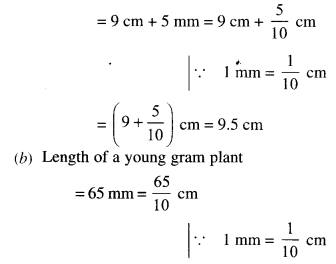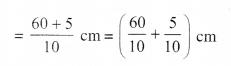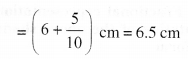We hope the NCERT Solutions for Class 6 Maths Chapter 8 Decimals Ex 8.1 help you. If you have any query regarding. NCERT Solutions for Class 6 Maths Chapter 8 Decimals Ex 8.1, drop a comment below and we will get back to you at the earliest.